GMAT  >  Test: Data Comparison

# Test: Data Comparison

Test Description

## 10 Questions MCQ Test Integrated Reasoning for GMAT | Test: Data Comparison

Test: Data Comparison for GMAT 2022 is part of Integrated Reasoning for GMAT preparation. The Test: Data Comparison questions and answers have been prepared according to the GMAT exam syllabus.The Test: Data Comparison MCQs are made for GMAT 2022 Exam. Find important definitions, questions, notes, meanings, examples, exercises, MCQs and online tests for Test: Data Comparison below.
Solutions of Test: Data Comparison questions in English are available as part of our Integrated Reasoning for GMAT for GMAT & Test: Data Comparison solutions in Hindi for Integrated Reasoning for GMAT course. Download more important topics, notes, lectures and mock test series for GMAT Exam by signing up for free. Attempt Test: Data Comparison | 10 questions in 20 minutes | Mock test for GMAT preparation | Free important questions MCQ to study Integrated Reasoning for GMAT for GMAT Exam | Download free PDF with solutions
 1 Crore+ students have signed up on EduRev. Have you?
Test: Data Comparison - Question 1

### k is a positive integer. v = 10 × k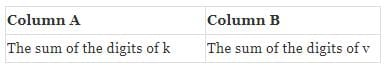Detailed Solution for Test: Data Comparison - Question 1

v = 10 x k ,
Let k = 325 , v = 3250,
The sum of the digits for both remains same which is = (3 + 2 + 5 + 0) = 10

Test: Data Comparison - Question 2

### m and n are integers. 0 < m < n < 10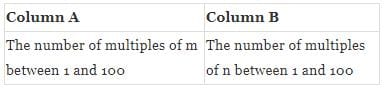Detailed Solution for Test: Data Comparison - Question 2

When m, n are integers between 1 and 10, and m < n, the number of multiples of m will be more than the number of multiples of n. E.g. for 8 , 9, the number of  multiples are respectively  12 and 11

Test: Data Comparison - Question 3

###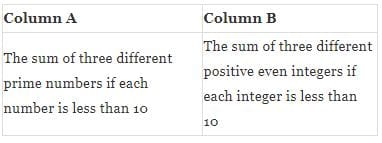Detailed Solution for Test: Data Comparison - Question 3

The max value of column A is 15 and min is 10,
The max value of column B is 18 and min is 12.
Hence, no relation can be found.

Test: Data Comparison - Question 4

a is a positive integer.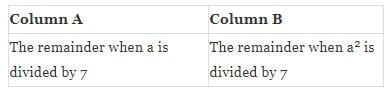Detailed Solution for Test: Data Comparison - Question 4

When a = 14, the remainder of a and a2 are respectively 0 and 0.
But when a = 16, the remainder of a and a2 are respectively 2 and 4.

Test: Data Comparison - Question 5

S = (-3, -2, 2, 3). The members of a set T are the squares of numbers in set S.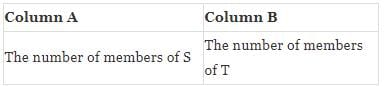Detailed Solution for Test: Data Comparison - Question 5

S = { -3 , -2 , 2 ,3 },  T = {9 , 4 }, n ( S ) = 4, n (T ) =2

Test: Data Comparison - Question 6

The average (arithmetic mean) of 5 positive integers is 70.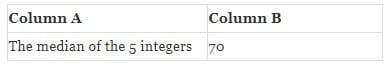Detailed Solution for Test: Data Comparison - Question 6

If the integers are (70 ,70 ,70, 70, 70) the median is 70, but if the integers are (60, 65, 65, 80, 80), the median is 65.

Test: Data Comparison - Question 7

R, S and T are 3 consecutive odd integers and R < S < T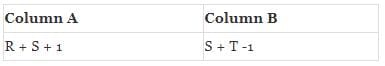Detailed Solution for Test: Data Comparison - Question 7

Since, they are consecutive odd numbers and R < S < T,  (S – R) = 2,    (T – S)  = 2, Adding (S + T)  – (R + S) = 4
⇒(S + T) =  ( R + S ) + 4,
⇒( S + T ) - 1 = ( R + S )  + 3  > ( R + S ) + 1

Test: Data Comparison - Question 8

x, y and z are negative integers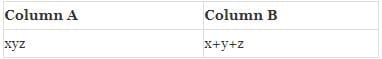Detailed Solution for Test: Data Comparison - Question 8

For x = -2, y = -3, z = – 4, x × y × z = -24,   x + y + z  = -9
But for x = y = z = -1, x × y × z = -1, x + y + z  = -3

Test: Data Comparison - Question 9

x is an integer, and the remainder when 2x is divided by 4 is 0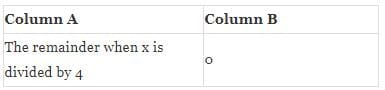Detailed Solution for Test: Data Comparison - Question 9

If x = 4, 2x = 8, 8 / 4,
the remainder is = 0.
Also for 4/4, the remainder is = 0.
But  if x = 6 ,2x = 12 , 12 / 4,
the remainder is = 0. But for 6 / 4 , the remainder is ≠ 0.

Test: Data Comparison - Question 10

Each of w and x is less than 5 and greater than 2. Each of y and z is less than 2 and greater than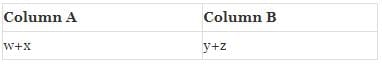Detailed Solution for Test: Data Comparison - Question 10

2 < w < 5, 2 < x < 5.
Adding, we get 4 < ( x + w ) < 10, 1 < y < 2, 1 < z < 2,
Adding, we get 2 < ( y + z ) < 4 < ( w + x ) < 10

## Integrated Reasoning for GMAT

19 videos|28 docs|24 tests
 Use Code STAYHOME200 and get INR 200 additional OFF Use Coupon Code
Information about Test: Data Comparison Page
In this test you can find the Exam questions for Test: Data Comparison solved & explained in the simplest way possible. Besides giving Questions and answers for Test: Data Comparison, EduRev gives you an ample number of Online tests for practice

## Integrated Reasoning for GMAT

19 videos|28 docs|24 tests